# Dynamic Programming on Trees | Set 2

Given a tree with N nodes and N-1 edges, find out the maximum height of tree when any node in the tree is considered as the root of the tree.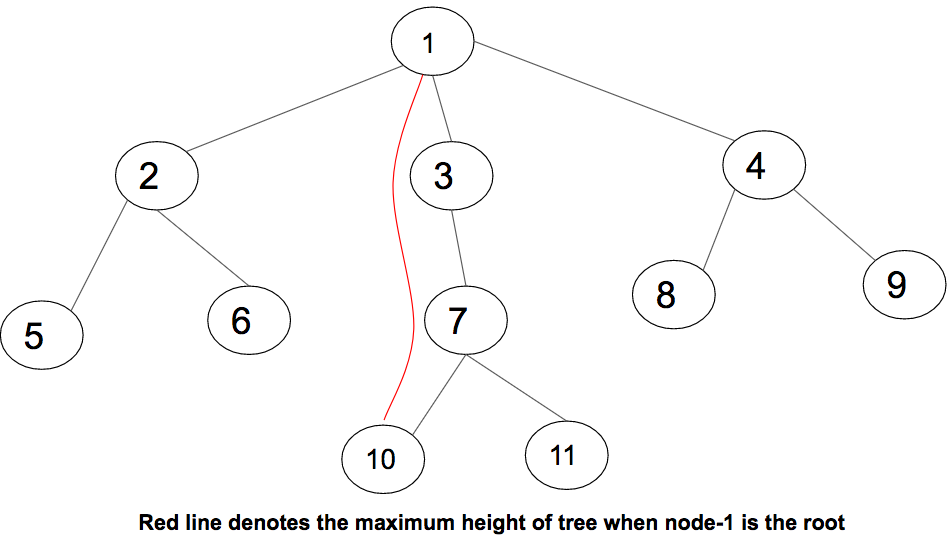The above diagram represents a tree with 11 nodes and 10 edges, and the path which gives us the maximum height when node 1 is considered as root. The maximum height is 3.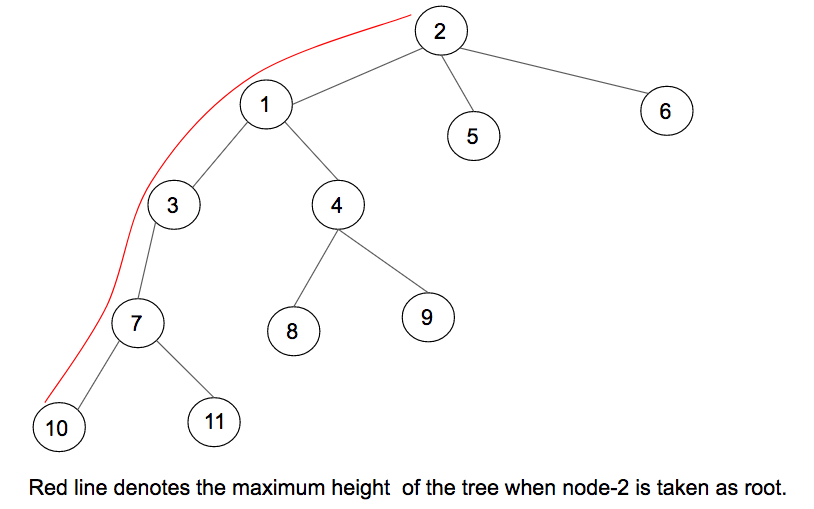In the above diagram, when 2 is considered as root, then the longest path found is in RED color. A naive approach will be to traverse the tree using DFS traversal for every node and calculate the maximum height when the node is treated as the root of the tree. The time complexity for DFS traversal of a tree is O(N). The overall time complexity of DFS for all N nodes will be O(N)*N i.e., O(N2).

The above problem can be solved by using Dynamic Programming on Trees. To solve this problem, pre-calculate two things for every node. One will be the maximum height while traveling downwards via its branches to the leaves. While the other will be the maximum height when traveling upwards via its parent to any of the leaves.

Optimal Substructure :
When node i is considered as root,
in[i] be the maximum height of tree when we travel downwards via its sub-trees and leaves.
Also, out[i] be the maximum height of the tree while traveling upwards via its parent.

The maximum height of tree when node i is
considered as root will be max(in[i], out[i]).

Calculation of in[i] :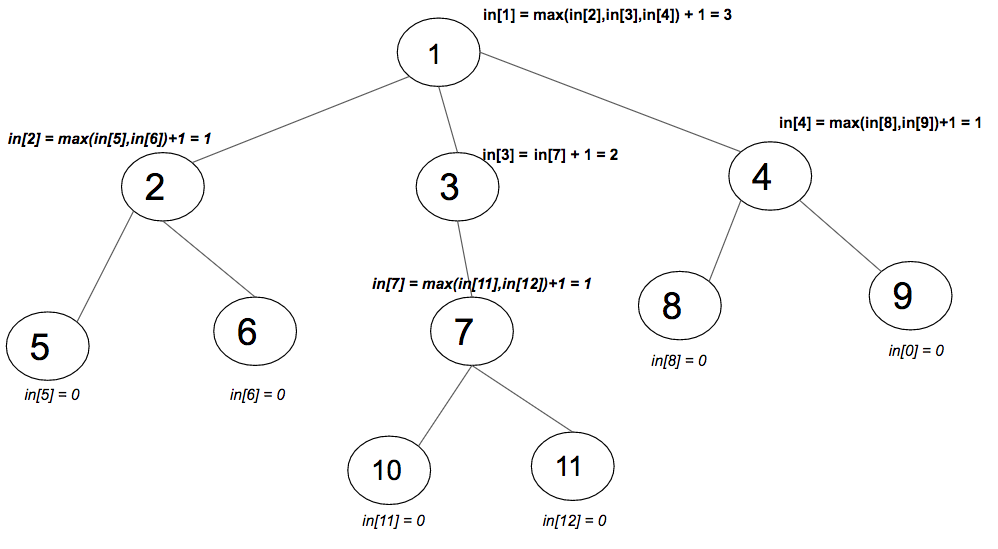In the image above, values of in[i] have been calculated for every node i. The maximum of every subtree is taken and added with 1 to the parent of that subtree. Add 1 for the edge between parent and subtree. Traverse the tree using DFS and calculate in[i] as max(in[i], 1+in[child]) for every node.
Calculation of out[i] :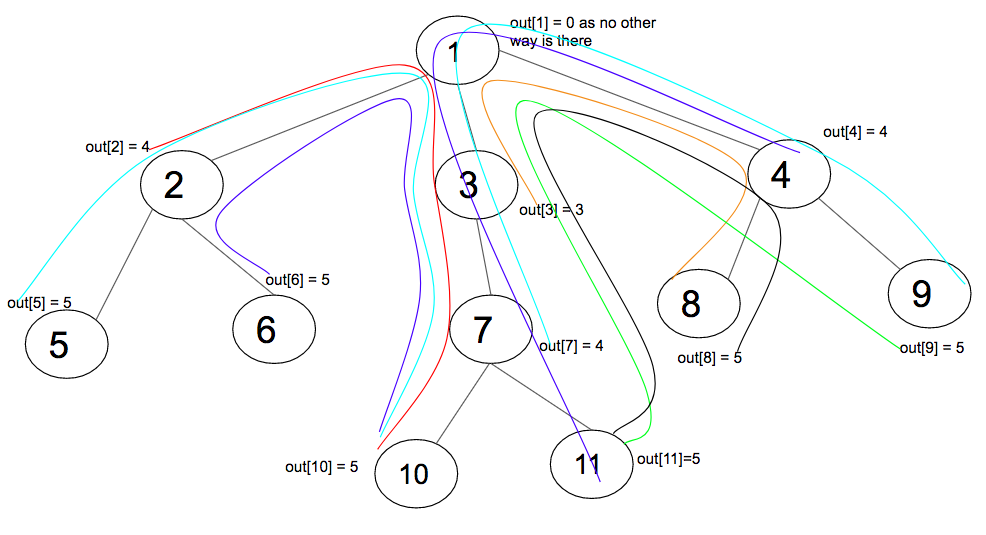The above diagram shows all the out[i] values and the path. For calculation of out[i], move upwards to the parent of node i. From the parent of node i, there are two ways to move in, one will be in all the branches of the parent. The other direction is to move to the parent(call it parent2 to avoid confusion) of the parent(call it parent1) of node i. The maximum height upwards via parent2 is out[parent1] itself. Generally, out[node i] as 1+max(out[i], 1+max of all branches). Add 1 for the edges between node and parent.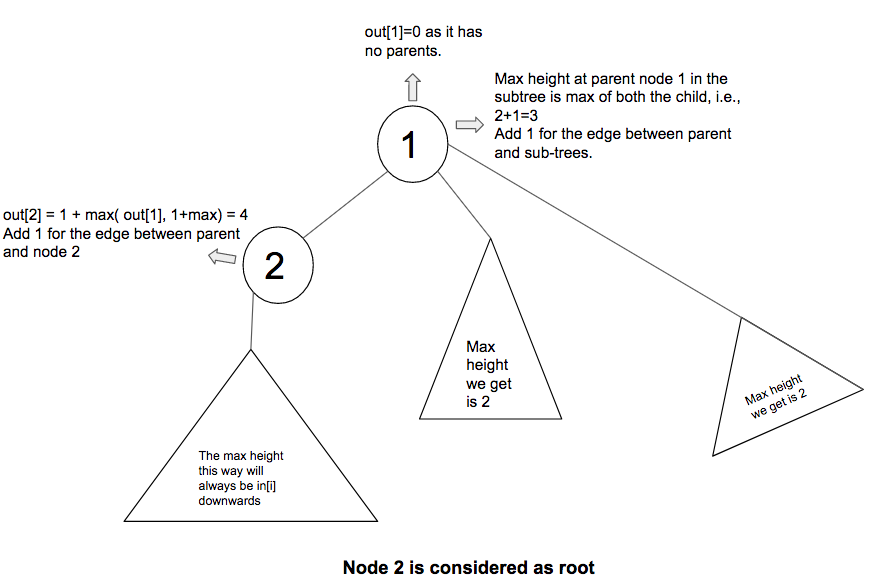The above diagram explains the calculation of out[i] when 2 is considered as the root of the tree. The branches of node 2 is not taken into count since the maximum height via that path has already been calculated and stored in i. Moving up, in this case, the parent of 2 i.e., 1 has no parent. So, the branches except for the one which has the node are considered while calculating the maximum.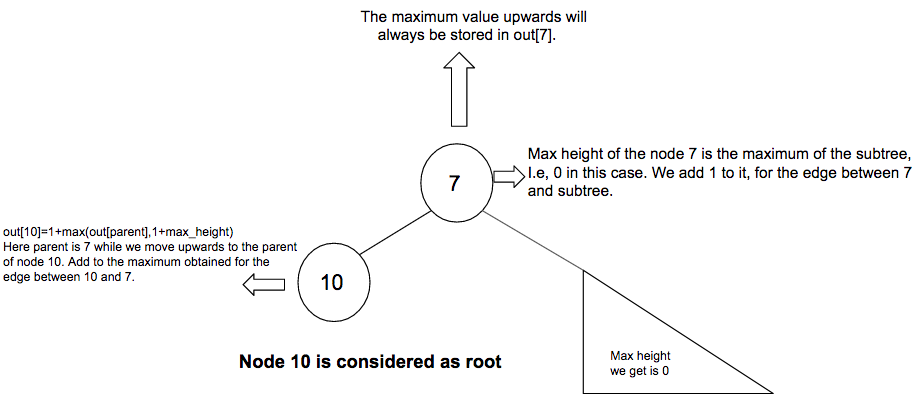The above diagram explains the calculation of out. The parent of node 10, i.e., 7 has a parent and a branch(precisely a child in this case). So the maximum height of both has been taken to count in such cases when parent and branches exist.
In case of multiple branches of a parent, take the longest of them to count(excluding the branch in which the node lies)

Calculating the maximum height of all the branches connected to parent :
in[i] stores the maximum height while moving downwards. No need to store all the lengths of branches. Only the first and second maximum length among all the branches will give answer. Since, algorithm used is based on DFS, all the branches connected to parent will be considered, including the branch which has the node. If the first maximum path thus obtained is same as in[i], then maximum1 is the length of the branch in which node i lies. In this case, our longest path will be maximum2.

Recurrence relation of in[i] and out[i] :

in[i] = max(in[i], 1 + in[child])
out[i] = 1 + max(out[parent of i], 1 + longest path of all branches of parent of i)

Below is the implementation of the above idea :

## C++

 `// C++ code to find the maximum path length ` `// considering any node as root ` `#include ` `using` `namespace` `std; ` `const` `int` `MAX_NODES = 100; ` ` `  `int` `in[MAX_NODES]; ` `int` `out[MAX_NODES]; ` ` `  `// function to pre-calculate the array in[] ` `// which stores the maximum height when travelled ` `// via branches ` `void` `dfs1(vector<``int``> v[], ``int` `u, ``int` `parent) ` `{ ` `    ``// initially every node has 0 height ` `    ``in[u] = 0; ` ` `  `    ``// traverse in the subtree of u ` `    ``for` `(``int` `child : v[u]) { ` ` `  `        ``// if child is same as parent ` `        ``if` `(child == parent) ` `            ``continue``; ` ` `  `        ``// dfs called ` `        ``dfs1(v, child, u); ` ` `  `        ``// recursively calculate the max height ` `        ``in[u] = max(in[u], 1 + in[child]); ` `    ``} ` `} ` ` `  `// function to pre-calculate the array ouut[] ` `// which stores the maximum height when traveled ` `// via parent ` `void` `dfs2(vector<``int``> v[], ``int` `u, ``int` `parent) ` `{ ` `    ``// stores the longest and second  ` `    ``// longest branches ` `    ``int` `mx1 = -1, mx2 = -1; ` ` `  `    ``// traverse in the subtress of u ` `    ``for` `(``int` `child : v[u]) { ` `        ``if` `(child == parent) ` `            ``continue``; ` ` `  `        ``// compare and store the longest ` `        ``// and second longest ` `        ``if` `(in[child] >= mx1) { ` `            ``mx2 = mx1; ` `            ``mx1 = in[child]; ` `        ``} ` ` `  `        ``else` `if` `(in[child] > mx2) ` `            ``mx2 = in[child]; ` `    ``} ` ` `  `    ``// traverse in the subtree of u ` `    ``for` `(``int` `child : v[u]) { ` `        ``if` `(child == parent) ` `            ``continue``; ` ` `  `        ``int` `longest = mx1; ` ` `  `        ``// if longest branch has the node, then ` `        ``// consider the second longest branch ` `        ``if` `(mx1 == in[child]) ` `            ``longest = mx2; ` ` `  `        ``// recursively calculate out[i] ` `        ``out[child] = 1 + max(out[u], 1 + longest); ` ` `  `        ``// dfs function call ` `        ``dfs2(v, child, u); ` `    ``} ` `} ` ` `  `// function to print all the maximum heights ` `// from every node ` `void` `printHeights(vector<``int``> v[], ``int` `n) ` `{ ` `    ``// traversal to calculate in[] array ` `    ``dfs1(v, 1, 0); ` ` `  `    ``// traversal to calculate out[] array ` `    ``dfs2(v, 1, 0); ` ` `  `    ``// print all maximum heights ` `    ``for` `(``int` `i = 1; i <= n; i++) ` `        ``cout << ``"The maximum height when node "`  `             ``<< i << ``" is considered as root"` `             ``<< ``" is "` `<< max(in[i], out[i])  ` `             ``<< ``"\n"``; ` `} ` ` `  `// Driver Code ` `int` `main() ` `{ ` `    ``int` `n = 11; ` `    ``vector<``int``> v[n + 1]; ` ` `  `    ``// initialize the tree given in the diagram ` `    ``v.push_back(2), v.push_back(1); ` `    ``v.push_back(3), v.push_back(1); ` `    ``v.push_back(4), v.push_back(1); ` `    ``v.push_back(5), v.push_back(2); ` `    ``v.push_back(6), v.push_back(2); ` `    ``v.push_back(7), v.push_back(3); ` `    ``v.push_back(10), v.push_back(7); ` `    ``v.push_back(11), v.push_back(7); ` `    ``v.push_back(8), v.push_back(4); ` `    ``v.push_back(9), v.push_back(4); ` ` `  `    ``// function to print the maximum height from every node ` `    ``printHeights(v, n); ` ` `  `    ``return` `0; ` `} `

## Java

 `// Java code to find the maximum path length ` `// considering any node as root ` `import` `java.io.*; ` `import` `java.util.*; ` ` `  `class` `GFG{ ` ` `  `static` `final` `int` `MAX_NODES = ``100``; ` `static` `int` `in[] = ``new` `int``[MAX_NODES]; ` `static` `int` `out[] = ``new` `int``[MAX_NODES]; ` ` `  `// Function to pre-calculate the array in[] ` `// which stores the maximum height when travelled ` `// via branches ` `static` `void` `dfs1(ArrayList> v, ` `                 ``int` `u, ``int` `parent) ` `{ ` `     `  `    ``// Initially every node has 0 height ` `    ``in[u] = ``0``; ` ` `  `    ``// Traverse in the subtree of u ` `    ``for``(``int` `j = ``0``; j < v.get(u).size(); j++)  ` `    ``{ ` `        ``int` `child = v.get(u).get(j); ` `         `  `        ``// If child is same as parent ` `        ``if` `(child == parent) ` `            ``continue``; ` ` `  `        ``// dfs called ` `        ``dfs1(v, child, u); ` ` `  `        ``// Recursively calculate the max height ` `        ``in[u] = Math.max(in[u], ``1` `+ in[child]); ` `    ``} ` `} ` ` `  `// Function to pre-calculate the array ouut[] ` `// which stores the maximum height when traveled ` `// via parent ` `static` `void` `dfs2(ArrayList> v, ` `                 ``int` `u, ``int` `parent) ` `{ ` `     `  `    ``// Stores the longest and second ` `    ``// longest branches ` `    ``int` `mx1 = -``1``, mx2 = -``1``; ` ` `  `    ``// Traverse in the subtress of u ` `    ``for``(``int` `j = ``0``; j < v.get(u).size(); j++) ` `    ``{ ` `        ``int` `child = v.get(u).get(j); ` `        ``if` `(child == parent) ` `            ``continue``; ` ` `  `        ``// Compare and store the longest ` `        ``// and second longest ` `        ``if` `(in[child] >= mx1)  ` `        ``{ ` `            ``mx2 = mx1; ` `            ``mx1 = in[child]; ` `        ``} ` ` `  `        ``else` `if` `(in[child] > mx2) ` `            ``mx2 = in[child]; ` `    ``} ` ` `  `    ``// Traverse in the subtree of u ` `    ``for``(``int` `j = ``0``; j < v.get(u).size(); j++) ` `    ``{ ` `        ``int` `child = v.get(u).get(j); ` `        ``if` `(child == parent) ` `            ``continue``; ` ` `  `        ``int` `longest = mx1; ` ` `  `        ``// If longest branch has the node, then ` `        ``// consider the second longest branch ` `        ``if` `(mx1 == in[child]) ` `            ``longest = mx2; ` ` `  `        ``// Recursively calculate out[i] ` `        ``out[child] = ``1` `+ Math.max(out[u], ``1` `+ longest); ` ` `  `        ``// dfs function call ` `        ``dfs2(v, child, u); ` `    ``} ` `} ` ` `  `static` `void` `addEdge(ArrayList> adj, ` `                    ``int` `u, ``int` `v) ` `{ ` `    ``adj.get(u).add(v); ` `    ``adj.get(v).add(u); ` `} ` ` `  `// Function to print all the maximum heights ` `// from every node ` `static` `void` `printHeights(ArrayList> v, ` `                         ``int` `n) ` `{ ` `     `  `    ``// Traversal to calculate in[] array ` `    ``dfs1(v, ``1``, ``0``); ` ` `  `    ``// Traversal to calculate out[] array ` `    ``dfs2(v, ``1``, ``0``); ` ` `  `    ``// Print all maximum heights ` `    ``for``(``int` `i = ``1``; i < n; i++) ` `        ``System.out.println( ` `            ``"The maximum height when node "` `+ i + ` `            ``" is considered as root is "` `+ ` `            ``Math.max(in[i], out[i])); ` `} ` ` `  `// Driver Code ` `public` `static` `void` `main(String[] args) ` `{ ` `     `  `    ``// Creating a graph with 11 vertices ` `    ``int` `V = ``12``; ` `    ``ArrayList> adj = ``new` `ArrayList>(V + ``1``); ` `    ``for``(``int` `i = ``0``; i < V; i++) ` `        ``adj.add(``new` `ArrayList()); ` ` `  `    ``// Initialize the tree given in the diagram ` `    ``addEdge(adj, ``1``, ``2``); ` `    ``addEdge(adj, ``1``, ``3``); ` `    ``addEdge(adj, ``1``, ``4``); ` `    ``addEdge(adj, ``2``, ``5``); ` `    ``addEdge(adj, ``2``, ``6``); ` `    ``addEdge(adj, ``3``, ``7``); ` `    ``addEdge(adj, ``7``, ``10``); ` `    ``addEdge(adj, ``7``, ``11``); ` `    ``addEdge(adj, ``4``, ``8``); ` `    ``addEdge(adj, ``4``, ``9``); ` ` `  `    ``// Function to print the maximum height ` `    ``// from every node ` `    ``printHeights(adj, V); ` `} ` `} ` ` `  `// This code is contributed by decoding `

Output :

```The maximum height when node 1 is considered as root is 3
The maximum height when node 2 is considered as root is 4
The maximum height when node 3 is considered as root is 3
The maximum height when node 4 is considered as root is 4
The maximum height when node 5 is considered as root is 5
The maximum height when node 6 is considered as root is 5
The maximum height when node 7 is considered as root is 4
The maximum height when node 8 is considered as root is 5
The maximum height when node 9 is considered as root is 5
The maximum height when node 10 is considered as root is 5
The maximum height when node 11 is considered as root is 5
```

Time Complexity : O(N)
Auxiliary Space : O(N)

Attention reader! Don’t stop learning now. Get hold of all the important DSA concepts with the DSA Self Paced Course at a student-friendly price and become industry ready.

My Personal Notes arrow_drop_upStriver(underscore)79 at Codechef and codeforces D

If you like GeeksforGeeks and would like to contribute, you can also write an article using contribute.geeksforgeeks.org or mail your article to contribute@geeksforgeeks.org. See your article appearing on the GeeksforGeeks main page and help other Geeks.

Please Improve this article if you find anything incorrect by clicking on the "Improve Article" button below.

Improved By : decoding, Akanksha_Rai